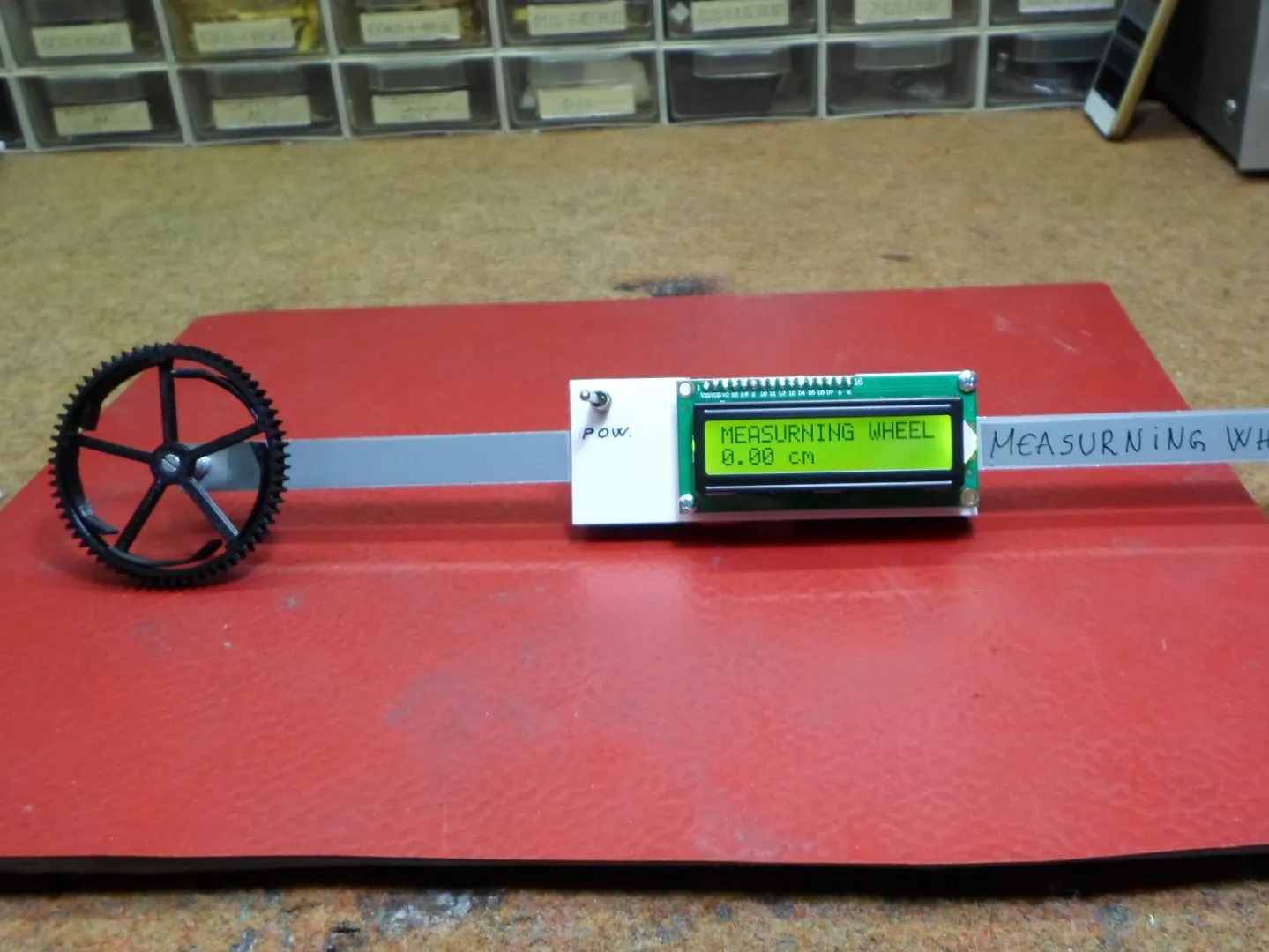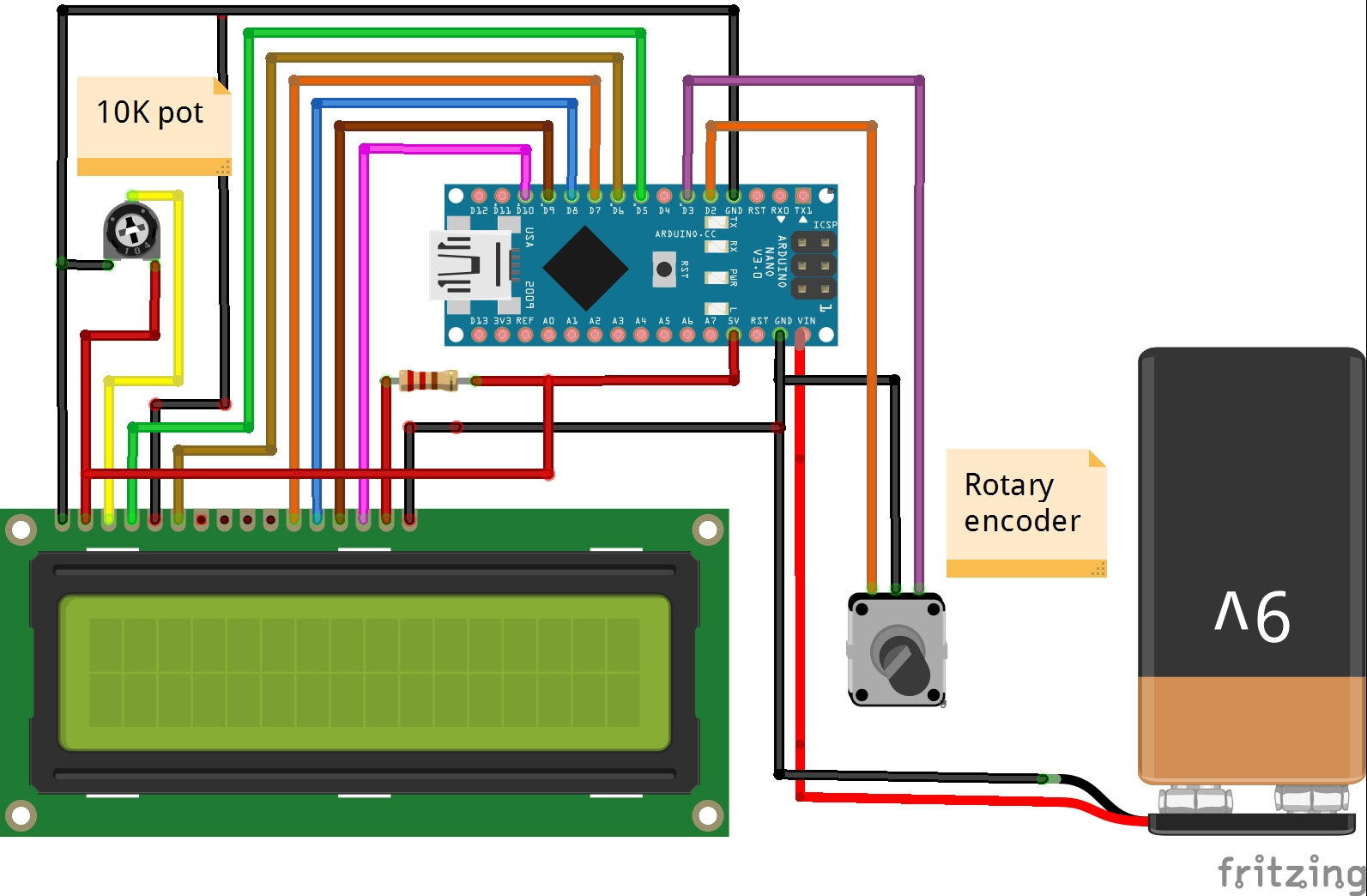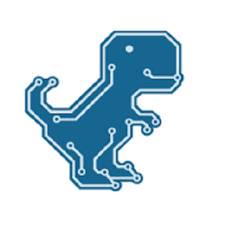Project tutorial# DIY Simple Measuring Wheel with Rotary Encoder © GPL3+

A useful tool for accurately measuring long distances.

• 19,175 views
• 23 respects

## Necessary tools and machinesSoldering iron (generic)

## Apps and online services

A measuring wheel is a construction measuring tool. The wheel rotates and, using basic rotational kinematics (s=rθ), you can determine the distance between two points.

The video below shows a reduced functional model of such a device made with several components:

- Arduino Nano

- Rotary encoder

- 16x2 LCD display

- 10k pot.

- 220 ohm resistor

The operating principle is as follows:

The rotary encoder measures the number of the rotation but we have to convert the rotation into travelled distance. Travelled distance depends on the diameter of the wheel. Rotary encoder moves N steps in one complete rotation (360 degree). Steps per rotation depends on the rotary encoder which can be changed from 8 to 48. Suppose N is the steps per rotation and R is the radius of wheel.

Travelled distance in one Rotation is = 2xπxR

Travelled distance in one Step is = 2xπxR/N

I wrote a very simple code for this purpose and the traveled distance is displayed on the LCD screen in centimeters. Depending on the components used in the code we change the values of "N" and "R".

In my case the wheel is made on a 3D printer and the whole assembly is mounted on an aluminum rod, as seen in the video.

## Code

##### CodeC/C++
```/*   Measurning Whell
*
*  by Mirko Pavleski,
*
*/

#include <LiquidCrystal.h>

LiquidCrystal lcd(5, 6, 7, 8, 9, 10);

int pin1 = 2;
int pin2 = 3;

int Pos = 0;
int State;
int LastState;

const float pi = 3.14;

const float R = 3.25;
const int N = 40;

float distance = 0;

void setup() {
pinMode (pin1 ,INPUT_PULLUP);
pinMode (pin2 ,INPUT_PULLUP);

lcd.begin(16, 2);
lcd.print("MEASURNING WHEEL");

}

void loop() {
if (State != LastState){
Pos ++;
}

else {
Pos --;
}
}

distance = ((2*pi*R)/N) * Pos ;

lcd.setCursor(0, 1);
lcd.print( distance);

lcd.setCursor(5, 1);
lcd.print("cm  ");

LastState = State;
}
```

## Schematics#### Author##### Mirko Pavleski
• 86 projects
• 474 followers

March 7, 2020

#### Members who respect this project

See similar projects
you might like

#### DIY Simple Frequency Meter Up to 6.5MHz

Project tutorial by Mirko Pavleski

• 12,320 views
• 19 respects

#### DIY Simple Square Wave Generator Up to 1MHz

Project tutorial by Mirko Pavleski

• 8,279 views
• 21 respects

#### DIY Autorange Ohmmeter

Project tutorial by Mirko Pavleski

• 6,306 views
• 1 comment
• 12 respects

#### DIY Simple Autorange Capacitance Meter (10pF-10000microF)

Project tutorial by Mirko Pavleski

• 10,868 views
• 20 respects

#### DIY Si4730 All Band Radio (LW, MW, SW, FM)

Project tutorial by Mirko Pavleski

• 51,026 views